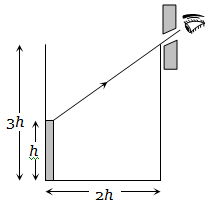An observer can see through a pin–hole the top end of a thin rod of height h, placed as shown in the figure. The beaker height is 3h and its radius h. When the beaker is filled with a liquid up to a height 2h, he can see the lower end of the rod. Then the refractive index of the liquid is(a) $5/2$                                    (b) $\sqrt{\left(5/2\right)}$

(c) $\sqrt{\left(3/2\right)}$                               (d) 3/2